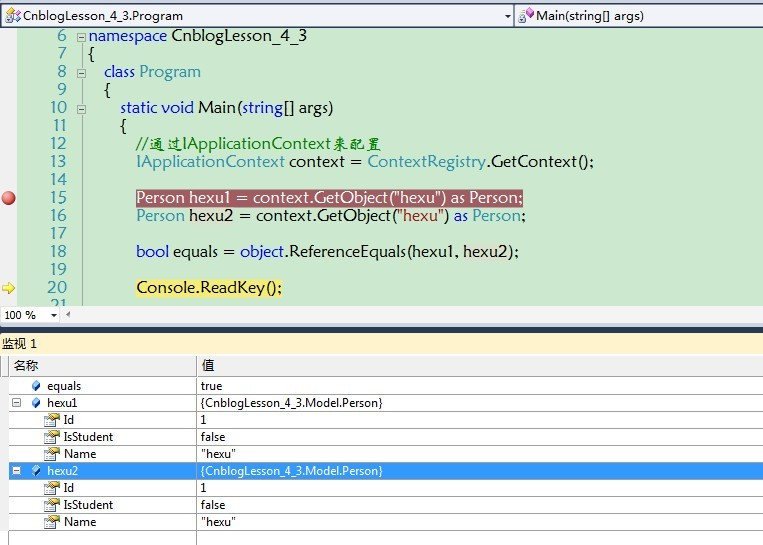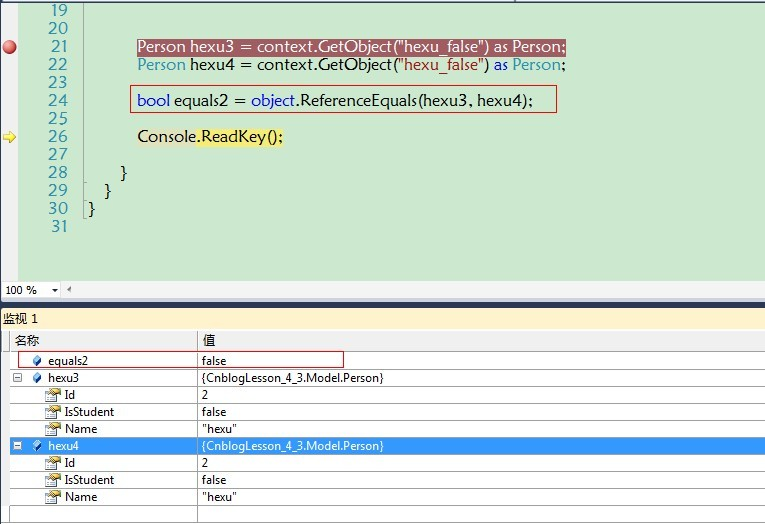## 第四章 Spring.Net 如何管理您的类___对象的作用域

Spring.Net 中对象的作用域，就是描述对象的部署模式 ，Spring.Net 中对象可以通过两种模式布署：

① singleton（单例模式）

② 非singleton 也叫非单例模式（或者叫做 prototype，只是用在这里不是很合适）

Objects.xml为:

1 <? xml version="1.0" encoding="utf-8" ?> 2 < objects xmlns ="http://www.springframework.net" > 3 4 <!-- 通过默认的 singleton="true" (默认为单例模式，我们这里显示设置) --> 5 < object id ="hexu" type ="CnblogLesson_4_3.Model.Person,CnblogLesson_4_3" singleton ="true" > 6 < property name ="Id" value ="1" /> 7 < property name ="Name" value ="hexu" /> 8 < property name ="IsStudent" value ="false" /> 9 </ object > 10 11 </ objects >Objects.xml为:

1 <? xml version="1.0" encoding="utf-8" ?> 2 < objects xmlns ="http://www.springframework.net" > 3 4 <!-- singleton="false" (设置为非单例模式) --> 5 < object id ="hexu_false" type ="CnblogLesson_4_3.Model.Person,CnblogLesson_4_3" singleton ="false" > 6 < property name ="Id" value ="2" /> 7 < property name ="Name" value ="hexu" /> 8 < property name ="IsStudent" value ="false" /> 9 </ object > 10 11 </ objects >